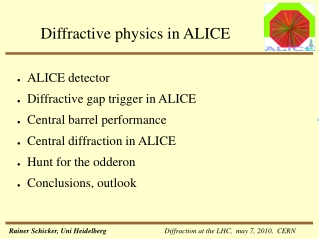# Diffractive physics in ALICE - PowerPoint PPT PresentationDownload PresentationDiffractive physics in ALICE

Diffractive physics in ALICE
Download Presentation## Diffractive physics in ALICE

- - - - - - - - - - - - - - - - - - - - - - - - - - - E N D - - - - - - - - - - - - - - - - - - - - - - - - - - -
##### Presentation Transcript

1. Diffractive physics in ALICE • ALICE detector • Diffractive gap trigger in ALICE • Central barrel performance • Central diffraction in ALICE • Hunt for the odderon • Conclusions, outlook

2. The ALICE experiment Acceptance central barrel -0.9 < h < 0.9 HMPID TRD MUON SPEC. ITS TPC Acceptance muon spectr. -2.5 < h < -4. TOF PHOS

3. ALICE diffractive gap trigger →additional forward detectors (no particle identification) 1 < h < 5 -4 < h < -1 → definition of gaps h+ , h_ p-p luminosity L = 5x1030cm-2s-1 : → one interaction/ 80 bunches diffractive L0 trigger (hardware): Pixel or TOF mult (central barrel) ADC ADA gap h+: 3 < h < 5 → Dh~ 0.5 gap h-: -2 < h < -4 → Dh~ 0.5 high level trigger (software): -3.7 < h < 5 → improved including ADA, ADD

4. ALICE central barrel comparison to other LHC detectors low magnetic field h-pt acceptance → low pT trigger ?

5. ALICE central barrel particle identification Particle identification by dE/dx in TPC as function of momentum Particle identification by time-of-flight information for non-relativistic momenta

6. ALICE central barrel electron/photon identification electron-pion separation in transition radiation detector at pT= 2 GeV/c Measure photon conversions by identifying e+e- in central barrel (V-topology) TRD L1 trigger on electrons →trigger on vector mesons J/Ψ, Υ by e+e- decay →identify π0, η at midrapidity

7. ALICE acceptance • ALICE acceptance matched to diffractive central production: double pomeron, (g –pomeron, odderon-pomeron) central barrel Dh ~ 3 Dh ~ 4 f • Data taking: • pp @ L = 5x1030 cm-2s-1 • pPb @ L = 1029 cm-2s-1 • PbPb @ L = 1027 cm-2s-1 gap had gap h

8. ALICE forward calorimeter • neutron calorimeter on each side • Placed at 116 m from interaction region • Measures neutral energy at 0o • Diffractive events with and without proton breakup: • pp → ppX : no energy in zero degree calorimeters • pp → pN*X, N*N*X : energy in one or in both calorimeters Identify the three topologies: B C A → what are f(xi), g(xi), h(xi) ?

9. ALICE 2010-2011: Central diffractive events Compare double gap events to min bias inelastic events of same multiplicity 1) Enhanced production cross section of glueball states: study resonances in central region when two rapidity gaps are required 2)Slope pomeron traj. a‘ ~ 0.25GeV-2 in DL fit, a‘ ~ 0.1GeV-2 in vector meson production at HERA, t-slope triple pom-vertex < 1GeV-2 → mean kt in pomeron wave function a‘ ~ 1/kt2 probably kt > 1 GeV →<pT> secondaries in double pomeron > <pT> secondaries min bias 3) kt > 1 GeV implies large effective temperature →K/p, h/p, h‘/p ratios enhanced

10. Central exclusive π+π- production at √s = 63 GeV Data taken by Axial Field Spectrometer at ISR √s = 63 GeV (R807) very forward drift chambers added for proton detection p π+π- p f0(600)=σ no ρ f0(980) 3500 events/25 MeV M(π+π-) G(1710)?? Structures not well understood beyond f(980) Not studied at higher √s T.Akesson et al 1986: Flavour independence: equal numbers of π+π- and K+K-pairs for masses larger than 1 GeV

11. χc as test of central exclusive production Formalism of central exclusive production predicts cross sections for γ γ, dijets, c,b Ingredients - unintegrated gluon distribution - cross section gg → X - Sudakov factor, no additional hard gluon - soft rescattering, suppression factor S2 ALICE: measure cwith rapidity gap on either side

12. dσexcl dy y=0 χc measurement χc: P-wave, spin triplet: • Harland-Lang,Khoze, Ryskin, Stirling 2010: = 45 nb →4.5x105χc0 in 106s c0 at LHC √s = 14 TeV: • in 106 s there are 90 χc0 proton-antiproton decays (4π) • Preliminary estimates:P. Ladron deGuevara et al: • estimates of efficiency reconstruction • PID cuts, solid angle ~ 32 % • → ~ 30 reconstructed in central barrel → detailed simulation studies ongoing

13. The hunt for the odderon • Diffractive J/Ψ production: contributions from • Pomeron-photon • Pomeron-odderon • Interference of photon-pomeron and photon-odderon amplitudes → different pT dist. of J/Ψ ds ~ Ag(AP + AO) 2 dNq ~ (AP2 + 2Re(APAO*) + AO2)dNq interference of C-even and C-odd amplitudes results in asymmetries of π+π- or K+K- pairs : → charge asymmetry relative to polar angle of π+ (K+) in dipion (dikaon) rest frame → asymmetries in HERA kinematics estimated 10% - 15% → how much are such asymmetries in pp @ LHC ?

14. Conclusions, outlook • ALICE has unique opportunity to do soft diffractive physics @LHC • Diffractive trigger defined by two rapidity gaps • Neutral energy measurement at 00 • Tests of central exclusive formalism at low masses • Phenomenology of Pomeron/Odderon • Photon-Photon physics